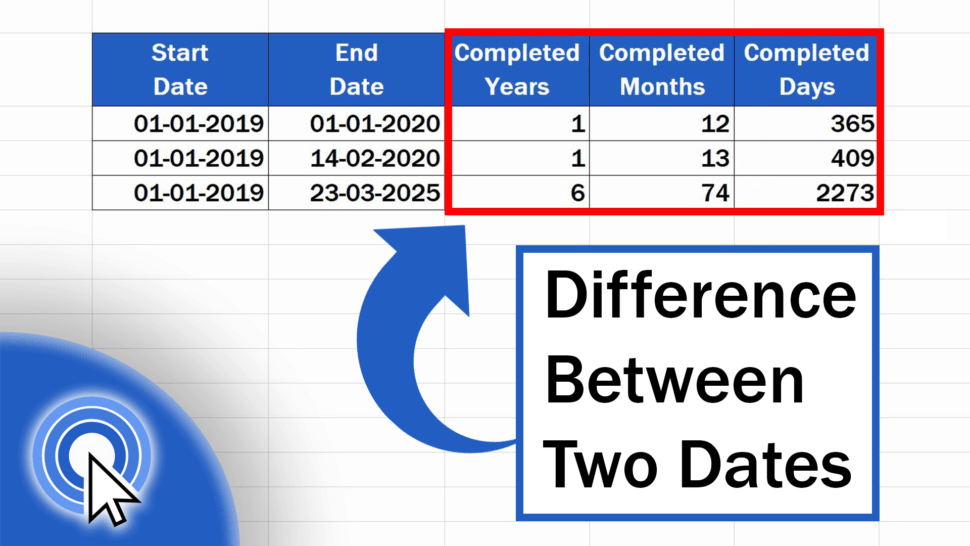# How to Calculate Difference Between Two Dates in ExcelThis tutorial shows how to calculate the difference between two dates in Excel. At the end, you’ll be able to conveniently use Excel formulas to figure out the exact number of years, months and days passed between two dates.

Let’s dive right into it!

See the video tutorial and transcription below:

Contents

Out of several possibilities, we’ll have a look at the easiest and quickest one to learn how to calculate the difference between two dates.

## Calculate Difference Between Two Dates in Excel Using the Function ‘DATE-DIF’

The most suitable function to do this kind of calculation is the function ‘DATE-DIF’. We’ll go through how it works right away.

First, click on the target cell where you want the result to appear. Type in the equal sign and carry on with typing the function ‘DATE-DIF’. Then enter the right data in the correct order into the brackets.

Begin with the ‘Start Date’, enter a comma and finish with the ‘End Date’.

But we’re not finished here. Enter another comma and right after it, add ‘Y’, which stands for ‘Years’. That must be done to signal that we want the exact number of completed years that passed between the two selected dates.

Close the brackets, press ‘Enter’ and ta-da – here’s the result!

## How to Copy the Function ‘DATE-DIF’ in Other Data Table Rows

This function can be ‘recycled’ very easily in our further calculations. Click on the bottom right-hand corner of the cell containing the formula and drag it through all the rows where we need the number of years calculated. Perfect!

Excel has calculated the number of completed years that passed between the dates in each row.

## How to Calculate How Many Months Passed between the Two Dates

But we still need to calculate the number of months and days.

So, let’s move on and see how many months and days passed between the two dates. We’ll use the same function, but we’ll need it slightly amended.

This is the little change we need to make to the formula.

Click again on the target cell and follow the steps you’ve just seen – type in the equal sign, then enter ‘DATE-DIF’, then the ‘Start Date’, a comma, the ‘End Date’, but now, instead of typing ‘Y’, type ‘M’ for ‘Months’, because we want to find out the number of completed months.

Close the brackets and the result appears right in front of us, in the target cell.

There’s no easier or quicker way to calculate the accurate number of months passed between two dates!

## How to Calculate How Many Days Passed between the Two Dates

And what do you think comes in the formula to calculate the number of completed days?

You’re about to find out whether you guessed right.

Start as usual – type in the equal sign, ‘DATE-DIF’, ‘Start Date’ and ‘End Date’ – all separated with a comma. And now we’ll finish the formula with ‘D’, for ‘Days’.

Close the brackets, hit ‘Enter’.

The number of completed days is as easy to figure out as the number of completed months or years.

This is a universal way to calculate the difference between two dates, working with any time data in a table.

Are you wondering:

If you found this tutorial helpful, give us a like and watch other video tutorials by EasyClick Academy. Learn how to use Excel in a quick and easy way!

Is this your first time on EasyClick? We’ll be more than happy to welcome you in our online community. Hit that Subscribe button and join the EasyClickers!

Thanks for watching and I’ll see you in the next tutorial!